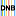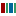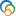The Library of Congress > Linked Data Service > LC Subject Headings (LCSH)

# Symmetry (Mathematics)

• ### Variants

• Invariance (Mathematics)

• ### Closely Matching Concepts from Other Schemes

•Invarianz•symmetryLabel from public data source Wikidata
•Symmetry (Mathematics)• ### Sources

• found: Work cat.: Ronan, Mark. Symmetry and the monster, 2006.
• found: CRC concise encyclopedia of mathematics, c2003:p. 2923 (Symmetry: an intrinsic property of a mathematical object which causes it to remain invariant under certain classes of transformations (such as rotation, reflection, inversions, or more abstract operations). The mathematical study of symmetry is systematized and formalized in the extremely powerful and beautiful area of mathematics called group theory.)
• found: Dictionary.com, Feb. 16, 2006(Symmetry (Mathematics): an attribute of a shape or relation; exact correspondence of form on opposite sides of a dividing line or plane [syn: symmetricalness, correspondence, balance] [ant: asymmetry])
• found: Encyclopaedia of mathematics, v. 9, c1993:p.102 (Symmetry: an involutory orthogonal transformation that changes the orientation (an involutory transformation is a transformation that, when applied twice, yields the identity.)
• found: Wikipedia.org, Feb. 16, 2006(Symmetry in mathematics occurs not only in geometry, but also in other branches of mathematics. It is actually the same as invariance: the property that something does not change under a set of transformations. A function of n variables may be invariant under certain permutations of the variables. These permutations form a group, a symmetry group ... One studies the symmetry of a given object by collecting all the operations that leave the object unchanged. These operations form a group. For a geometrical object, this is known as its symmetry group; for an algebraic object, one uses the term automorphism group.)
• notfound: Encyclopedic dictionary of mathematics;On-line Mathematics Dictionary
• ### LC Classification

• QA174.7.S96
• ### Change Notes

• 2006-02-16: new
• 2006-04-14: revised

# Suggest terminology

The LC Linked Data Service welcomes any suggestions you might have about terminology used for a given heading or concept.

Would you like to suggest a change to this heading?

Please provide your name, email, and your suggestion so that we can begin assessing any terminology changes.

Fields denoted with an asterisk (*) are required.